Note: The other languages of the website are Google-translated. Back to English

## Excel Formula: Convert Excel Time to Unix Timestamp or vice inverse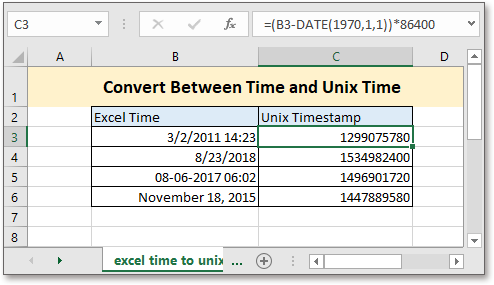In this essay, it talks about the formulas that convert between Excel time and Unix timestamp in Excel sheet.

What is Unix timestamp?

Unix timestamp records time by using seconds. In the Unix system, it counts time from January 1, 1970. In other words, the Unix timestamp is the total number of seconds between the given date and January 1, 1970.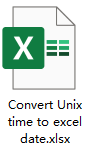### Convert Excel time to Unix timestamp

Generic formula:

 (Excel_time-DATE(1970,1,1))*86400

Syntaxt and Arguments

 Excel_time: the datetime that you want to convert to Unix time.

Return Value

The formula returns a series number. Usually the returned value is such big that it displays as #####, and you can format the returned value as General or Number to show it as a regular series number.

Remark

If the given Excel time is greater than or equal to Jan 1, 1900 but less than Jan 1, 1970, it returns a negative number; if the Excel time is equal to Jan 1, 1970, it returns zero; if the Excel time is greater than Jan 1, 1970, it returns a positive number.

Error

If the Excel time is less than Jan 1, 1900, the result returns an error value #VALUE!

How this formula work

Here to convert the Excel time in cell B3:B6 to Unix Time, please use below formula:

 =(B3-DATE(1970,1,1))*86400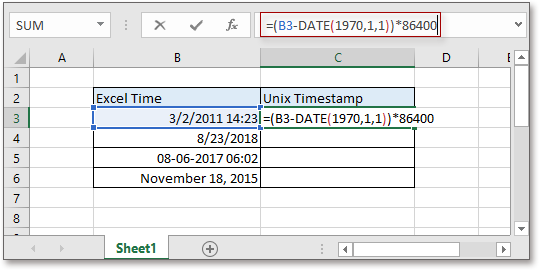Press Enter key to get the first result, then drag auto fill handle down to cell C7.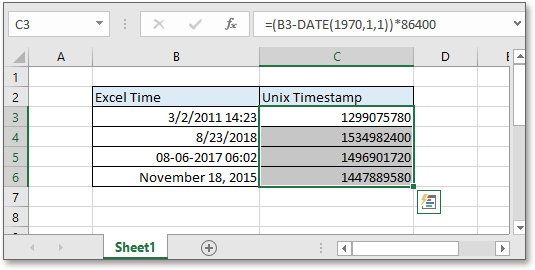Explanation

Date function: create a date with the year number, month number and day number.

The formula (B3-DATE(1970,1,1)) is used to calculate the difference between the given date time and the Unix epoch.

86400: As we known, one day = 24 hours, one hour = 60 minutes, and one minute = 60 seconds, so one day = 24*60*60=86400 seconds.

### Convert Unix timestamp to Excel time

Generic formula:

 (Unix_time/86400)+DATE(1970,1,1)

Syntaxt and Arguments

 Unix_time: the Unix time (a series number) that you want to convert to standard Excel time.

Return Value

The formula returns a series number, then you need to format the series number to a datetime formatting. Usually the result is a positive series number, if the result is a negative number, it will return to a series of ##### after formatting it as datetime.

How this formula work

To convert the Unix time in cell B3:B6 to Excel time, please use below formula:

 =(B3/86400)+DATE(1970,1,1)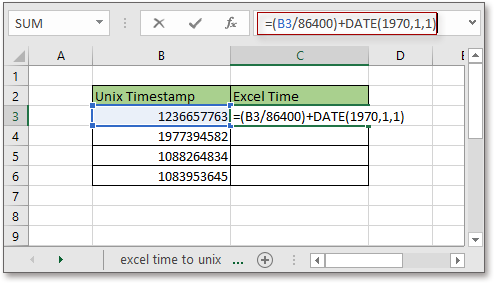Press Enter key and drag auto fill handle down to cell C6.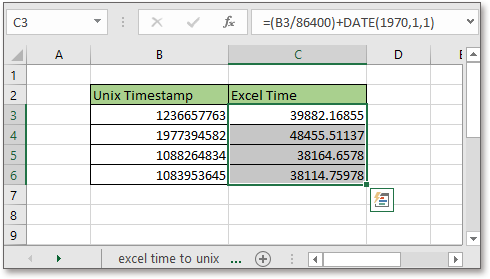Then keep the results selected, then press Ctrl + 1 to display the Format Cells dialog, under Number tab, select Date from the Category list, then in the right section of Type, choose one format you need.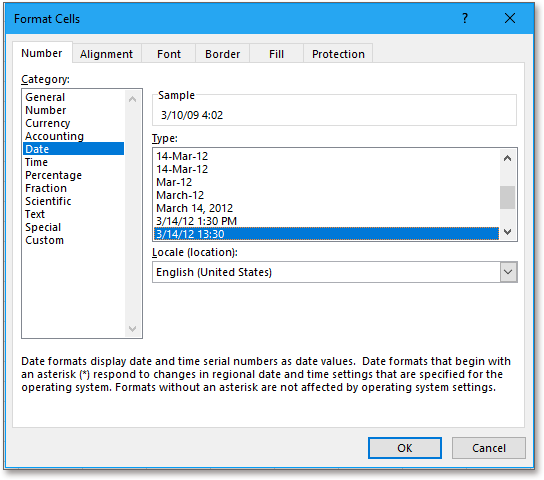Click OK then the formula results have been formatted as datetime.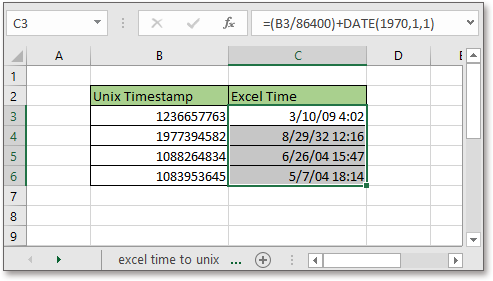Explanation

Date function: create a date with the year number, month number and day number.

The formula (B3/86400)+DATE(1970,1,1) gets the total number of days between the given date time and the Unix epoch.

86400: As we known, one day = 24 hours, one hour = 60 minutes, one minute = 60 seconds, so one day = 24*60*60=86400 seconds. And vice versa, a date/86400 will get the day number.

### The Best Office Productivity Tools

#### Kutools for Excel - Helps You To Stand Out From Crowd

Would you like to complete your daily work quickly and perfectly? Kutools for Excel brings 300 powerful advanced features (Combine workbooks, sum by color, split cell contents, convert date, and so on...) and save 80% time for you.

• Designed for 1500 work scenarios, helps you solve 80% Excel problems.
• Reduce thousands of keyboard and mouse clicks every day, relieve your tired eyes and hands.
• Become an Excel expert in 3 minutes. No longer need to remember any painful formulas and VBA codes.
• 30-day unlimited free trial. 60-day money back guarantee. Free upgrade and support for 2 years.#### Office Tab - Enable Tabbed Reading and Editing in Microsoft Office (include Excel)

• One second to switch between dozens of open documents!
• Reduce hundreds of mouse clicks for you every day, say goodbye to mouse hand.
• Increases your productivity by 50% when viewing and editing multiple documents.
• Brings Efficient Tabs to Office (include Excel), Just Like Chrome, Firefox, And New Internet Explorer.